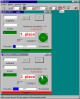Calcul 5.2.WIN32 CALCUL to practise mental arithmetic’s or fractions. Freeware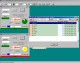CalculPro 1.2.1 CalculPro to practise mental arithmetic’s or fractions. Shareware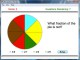FRS Fraction Master 2.1 Learn fractions faster. Shareware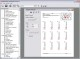Mathematics Worksheet Factory 3.1 A powerful, easy-to-use math worksheet generation program. Shareware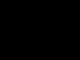Math Resource Studio 4.4.2 A powerful, easy-to-use math worksheet generation program. DemoAbilities Builder Fraction Facts 3.5 Build fraction reducing and simplifying skills for simple to complex problems. Demo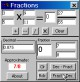Fractions n Decimals CE 5.3 Add, Subtract, Divide and Multiply fractions. Convert decimals/fractions CE 2.11 SharewareAbilities Builder Fractions Plus 1.0 Add, subtract, multiply and divide simple to complex fraction problems. Demo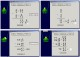Abilities Builder Add & Subtract Fractions 3.5 Build fraction addition and subtraction skills for simple to complex problems. Demo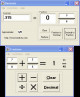Breaktru Fractions n Decimals 10.2 Add, Subtract, Divide and Multiply fractions. Convert decimals / fractions Shareware